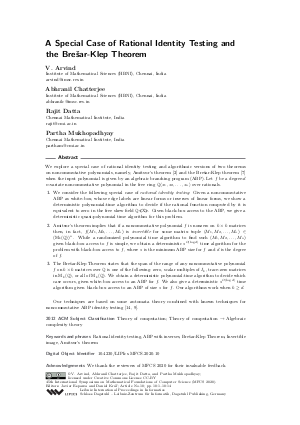Document# A Special Case of Rational Identity Testing and the Brešar-Klep Theorem

### Authors V. Arvind, Abhranil Chatterjee, Rajit Datta, Partha Mukhopadhyay## File

LIPIcs.MFCS.2020.10.pdf
• Filesize: 0.49 MB
• 14 pages

## Acknowledgements

We thank the reviewers of MFCS 2020 for their invaluable feedback.

## Cite As

V. Arvind, Abhranil Chatterjee, Rajit Datta, and Partha Mukhopadhyay. A Special Case of Rational Identity Testing and the Brešar-Klep Theorem. In 45th International Symposium on Mathematical Foundations of Computer Science (MFCS 2020). Leibniz International Proceedings in Informatics (LIPIcs), Volume 170, pp. 10:1-10:14, Schloss Dagstuhl - Leibniz-Zentrum für Informatik (2020)
https://doi.org/10.4230/LIPIcs.MFCS.2020.10

## Abstract

We explore a special case of rational identity testing and algorithmic versions of two theorems on noncommutative polynomials, namely, Amitsur's theorem [S.A Amitsur, 1966] and the Brešar-Klep theorem [Brešar and Klep, 2008] when the input polynomial is given by an algebraic branching program (ABP). Let f be a degree-d n-variate noncommutative polynomial in the free ring Q<x_1,x_2,...,x_n> over rationals. 1) We consider the following special case of rational identity testing: Given a noncommutative ABP as white-box, whose edge labels are linear forms or inverses of linear forms, we show a deterministic polynomial-time algorithm to decide if the rational function computed by it is equivalent to zero in the free skew field Q<(X)>. Given black-box access to the ABP, we give a deterministic quasi-polynomial time algorithm for this problem. 2) Amitsur's theorem implies that if a noncommutative polynomial f is nonzero on k x k matrices then, in fact, f(M_1,M_2,...,M_n) is invertible for some matrix tuple (M_1,M_2,...,M_n) in (M_k(ℚ))^n. While a randomized polynomial time algorithm to find such (M_1,M_2,...,M_n) given black-box access to f is simple, we obtain a deterministic s^{O(log d)} time algorithm for the problem with black-box access to f, where s is the minimum ABP size for f and d is the degree of f. 3) The Brešar-Klep Theorem states that the span of the range of any noncommutative polynomial f on k x k matrices over Q is one of the following: zero, scalar multiples of I_k, trace-zero matrices in M_k(Q), or all of M_k(Q). We obtain a deterministic polynomial-time algorithm to decide which case occurs, given white-box access to an ABP for f. We also give a deterministic s^{O(log d)} time algorithm given black-box access to an ABP of size s for f. Our algorithms work when k >= d. Our techniques are based on some automata theory combined with known techniques for noncommutative ABP identity testing [Ran Raz and Amir Shpilka, 2005; Michael A. Forbes and Amir Shpilka, 2013].

## Subject Classification

##### ACM Subject Classification
• Theory of computation
• Theory of computation → Algebraic complexity theory
##### Keywords
• Rational identity testing
• ABP with inverses
• Brešar-Klep Theorem
• Invertible image
• Amitsur’s theorem

## Metrics

• Access Statistics
• Total Accesses (updated on a weekly basis)
0

## References

1. A. S. Amitsur and J. Levitzki. Minimal identities for algebras. Proceedings of the American Mathematical Society, 1(4):449-463, 1950. URL: http://www.jstor.org/stable/2032312.
2. S.A Amitsur. Rational identities and applications to algebra and geometry. Journal of Algebra, 3(3):304-359, 1966. URL: https://doi.org/10.1016/0021-8693(66)90004-4.
3. Vikraman Arvind, Abhranil Chatterjee, Rajit Datta, and Partha Mukhopadhyay. Efficient black-box identity testing for free group algebras. In Approximation, Randomization, and Combinatorial Optimization. Algorithms and Techniques, APPROX/RANDOM 2019, September 20-22, 2019, Massachusetts Institute of Technology, Cambridge, MA, USA, pages 57:1-57:16, 2019. URL: https://doi.org/10.4230/LIPIcs.APPROX-RANDOM.2019.57.
4. Vikraman Arvind, Partha Mukhopadhyay, and Srikanth Srinivasan. New results on noncommutative and commutative polynomial identity testing. Computational Complexity, 19(4):521-558, 2010. URL: https://doi.org/10.1007/s00037-010-0299-8.
5. J. Berstel and C. Reutenauer. Noncommutative Rational Series with Applications. Encyclopedia of Mathematics and its Applications. Cambridge University Press, 2011. URL: https://books.google.co.in/books?id=LL8Nhn72I_8C.
6. Andrej Bogdanov and Hoeteck Wee. More on noncommutative polynomial identity testing. In 20th Annual IEEE Conference on Computational Complexity (CCC 2005), 11-15 June 2005, San Jose, CA, USA, pages 92-99, 2005. URL: https://doi.org/10.1109/CCC.2005.13.
7. Matej Brešar and Igor Klep. Values of noncommutative polynomials, lie skew-ideals and the tracial nullstellensatz. Mathematical Research Letters, 2008.8. Harm Derksen and Visu Makam. Polynomial degree bounds for matrix semi-invariants. Advances in Mathematics, 310:44-63, 2017. URL: https://doi.org/10.1016/j.aim.2017.01.018.
9. Michael A. Forbes and Amir Shpilka. Quasipolynomial-time identity testing of non-commutative and read-once oblivious algebraic branching programs. In 54th Annual IEEE Symposium on Foundations of Computer Science, FOCS 2013, 26-29 October, 2013, Berkeley, CA, USA, pages 243-252, 2013. URL: https://doi.org/10.1109/FOCS.2013.34.
10. Ankit Garg, Leonid Gurvits, Rafael Mendes de Oliveira, and Avi Wigderson. A deterministic polynomial time algorithm for non-commutative rational identity testing. 2016 IEEE 57th Annual Symposium on Foundations of Computer Science (FOCS), pages 109-117, 2016.11. Pavel Hrubeš and Avi Wigderson. Non-commutative arithmetic circuits with division. Theory of Computing, 11(14):357-393, 2015. URL: https://doi.org/10.4086/toc.2015.v011a014.
12. Gábor Ivanyos, Youming Qiao, and K. V. Subrahmanyam. Constructive non-commutative rank computation is in deterministic polynomial time. computational complexity, 27(4):561-593, December 2018. URL: https://doi.org/10.1007/s00037-018-0165-7.
13. Sylvain Lombardy and Jacques Sakarovitch. The removal of weighted ε-transitions. In Nelma Moreira and Rogério Reis, editors, Implementation and Application of Automata, pages 345-352, Berlin, Heidelberg, 2012. Springer Berlin Heidelberg.14. Ran Raz and Amir Shpilka. Deterministic polynomial identity testing in non-commutative models. Computational Complexity, 14(1):1-19, 2005. URL: https://doi.org/10.1007/s00037-005-0188-8.
15. M.P. Schützenberger. On the definition of a family of automata. Information and Control, 4(2):245-270, 1961. URL: https://doi.org/10.1016/S0019-9958(61)80020-X.
16. Dirk Werner. Funktionalanalysis (in German). Springer Verlag, 2005.X

Feedback for Dagstuhl Publishing